﻿﻿﻿﻿﻿ Proven to be the world | Scholastic: Children Book Publishing

### Proven to be the world's best practice

ALPHA Mathematics is a world-class program based on the effective teaching and learning practices of Singapore, Republic of Korea and Hong Kong - consistent top performers in international studies.

Trends in International Mathematics and Science Study (TIMSS) is an international study that evaluates the skills and knowledge of Grade 4 and Grade 8 students.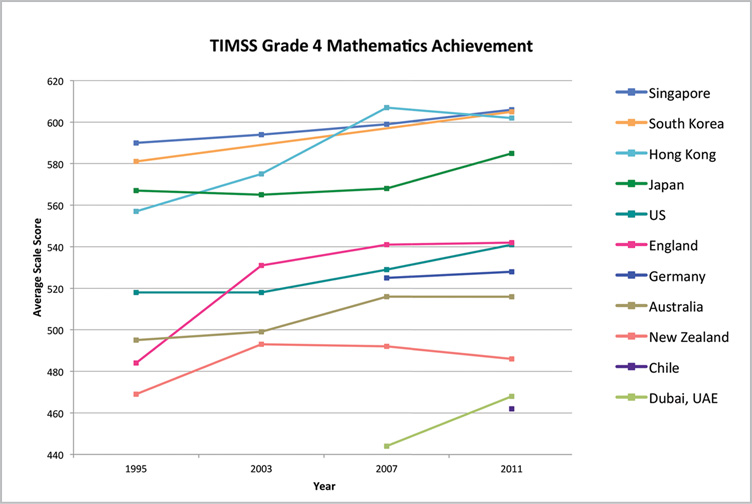Programme for International Student Assessment (PISA) is an international study that evaluates the skills and knowledge of 15-year-old students.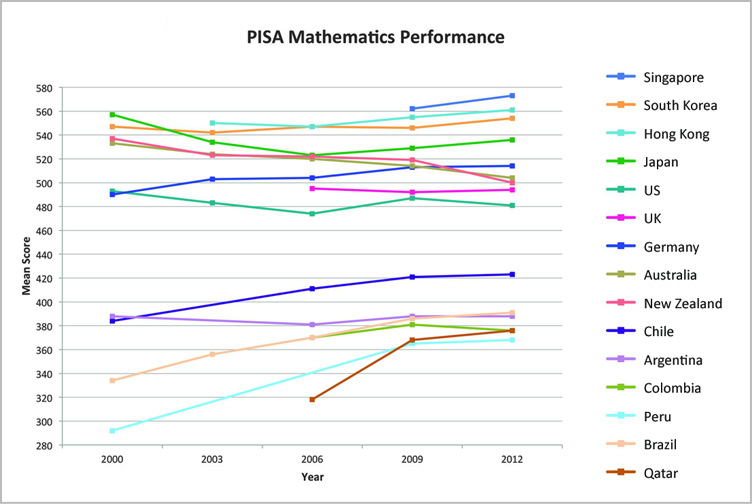### How Do Global Top Performers Do It?

Global top-performing nations have much in common in their vision for mathematics teaching and learning, expectations of outcomes and pedagogical approach and practices.

 Teaching is for learning; learning is for understanding; understanding is for reasoning and applying and, ultimately problem solving" Curricular emphasis is on the understanding of fundamental mathematical concepts or principles, logical thinking, problem solving, communication, and mathematical dispositions." Students should be able to understand thoroughly what they have learnt, master problem solving confidently and develop a positive learning attitude." - Ministry of Education, Singapore - Korea National University of Education - Education Department, Hong Kong Special Administrative Region

### Effective Teaching and Learning Practices of Top-Performing Nations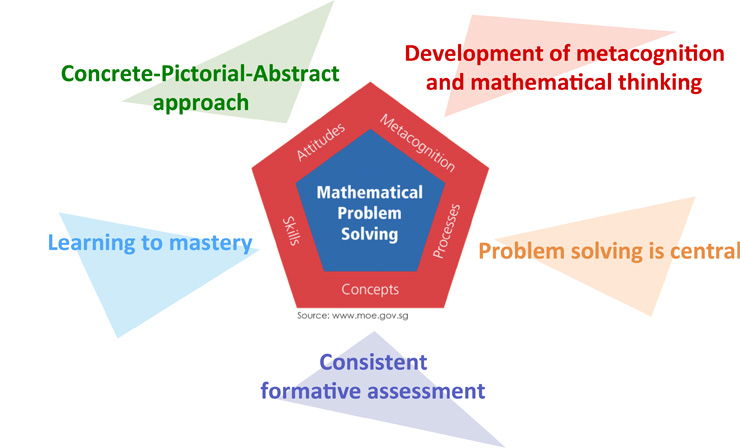#### Problem Solving Is Central

• Developing problem solving skills should address both the process and the method of solving problems.
• Students learn to use different strategies and solve problems effectively and confidently.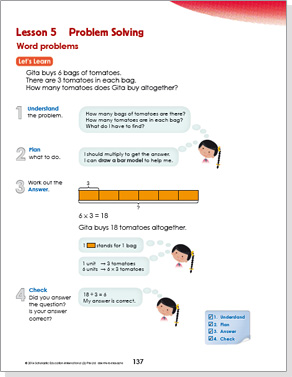#### Concrete-Pictorial-Abstract Approach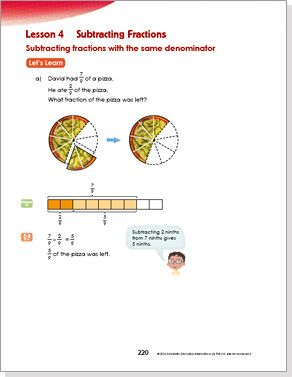• The Concrete-Pictorial-Abstract Approach develops deep conceptual understanding.
• Students learn to make connections between physical materials, visual representations and mathematical symbols.

#### Development of Metacognition and Mathematical Thinking

• Thinking mathematically is a conscious habit.
• Students learn to monitor, direct and communicate their thought processes and mathematical thinking.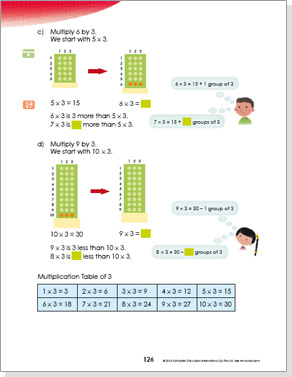The main goal of mathematics education in schools is the mathematisation of the child's thinking. Clarity of thought and pursuing assumptions to logical conclusions is central to the mathematical enterprise. There are many ways of thinking, and the kind of thinking one learns in mathematics is an ability to handle abstractions, and an approach to problem solving." - Position Paper, National Focus Group on Teaching of Mathematics, NCERT

#### Learning to Mastery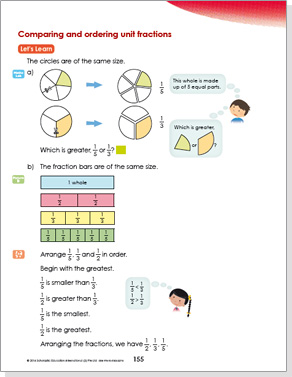• Learning to mastery involves concept development and understanding mathematical relationships.
• Students learn to inquire, communicate, reason, conceptualise, formulate and solve problems.

#### Consistent Formative Assessment

• Assessment is a routine part of the on-going classroom activity.
• Students' understanding of a concept just taught should be assessed immediately to identify remediation needs.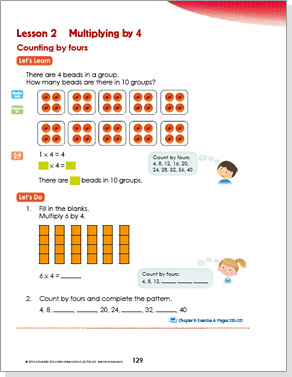### The Mathematics Framework

The central focus of the framework is mathematical problem solving. The five inter-related components of the framework are integral parts of mathematics learning and problem solving.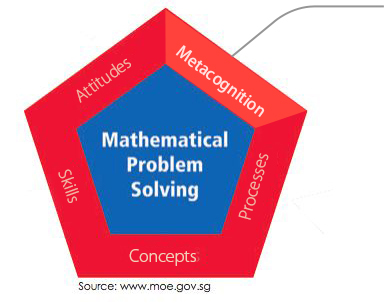###### Metacognition

Metacognition, or "thinking about thinking", refers to the awareness of, and the ability to control one's thinking processes, in particular the selection and use of problem-solving strategies.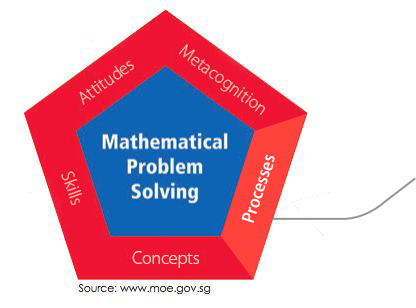###### Processes

Mathematical processes refer to the skills involved to acquire and apply mathematical knowledge. This includes reasoning, communication, thinking skills and heuristics, and application and modelling.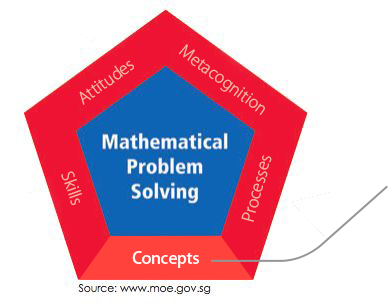###### Concepts

Mathematical concepts cover numerical, algebraic, geometrical, statistical, probabilistic, and analytical concepts. Students should develop the mathematics ideas in depth and as an integrated whole.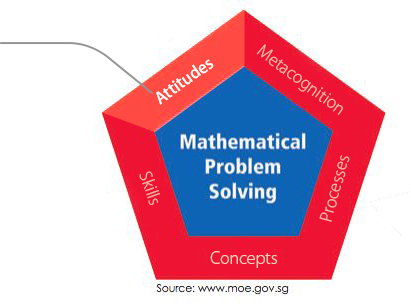###### Attitudes

Attiudes refer to the affective aspects of mathematics learning such as:

• Appreciation of mathematics and its usefulness
• Interest in learning mathematics
• Confidence in using mathematics
• Perseverance in solving a problem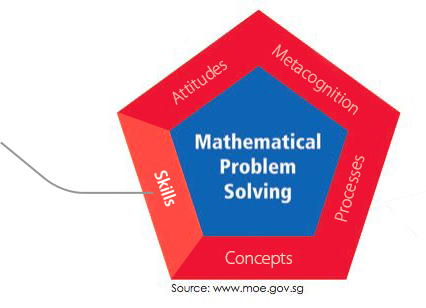###### Skills

Mathematical skills include procedural skills for numerical calculation, algebraic manipulation, spatial visualisation, data analysis, measurement, use of mathematical tools, and estimation.